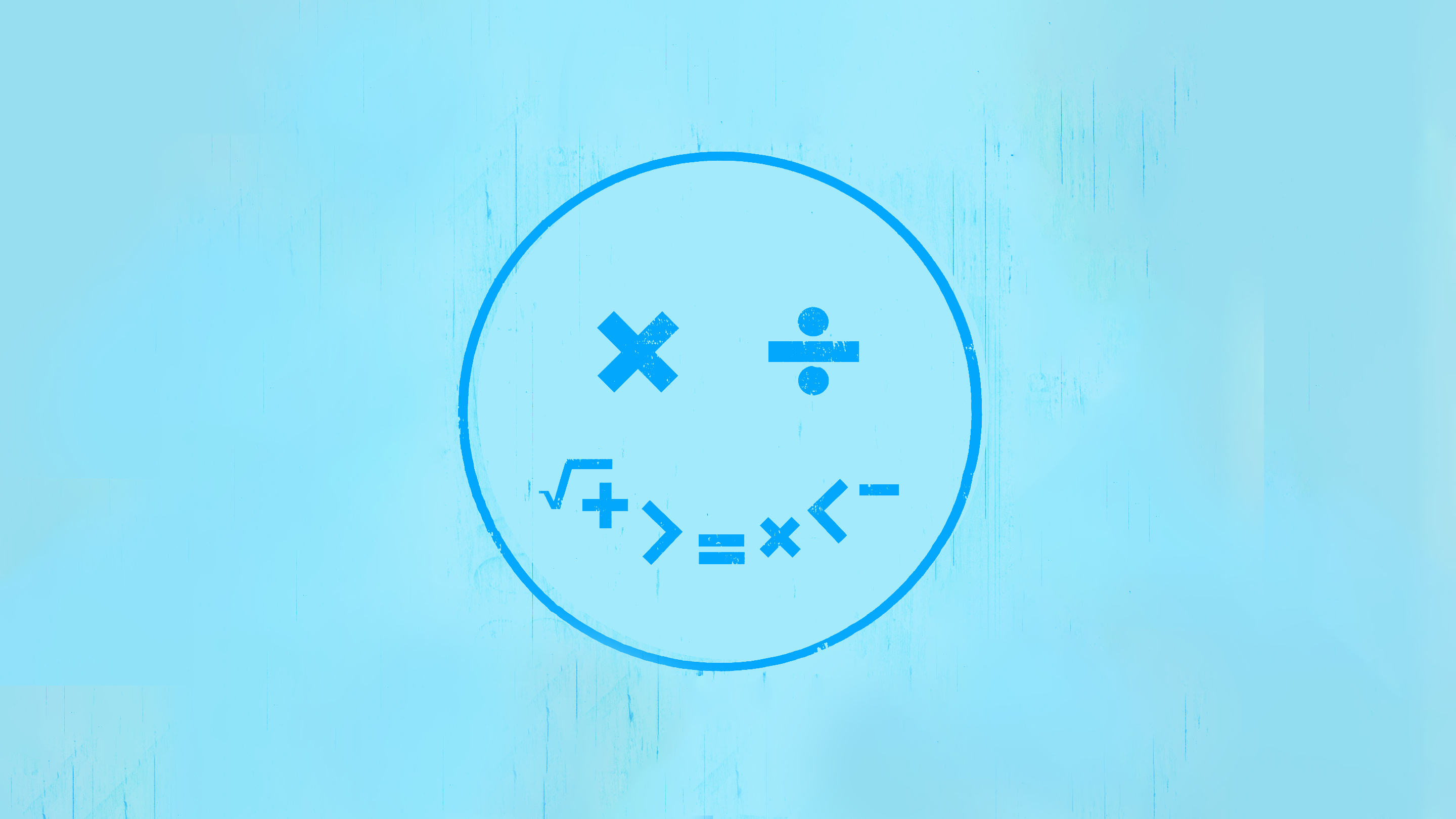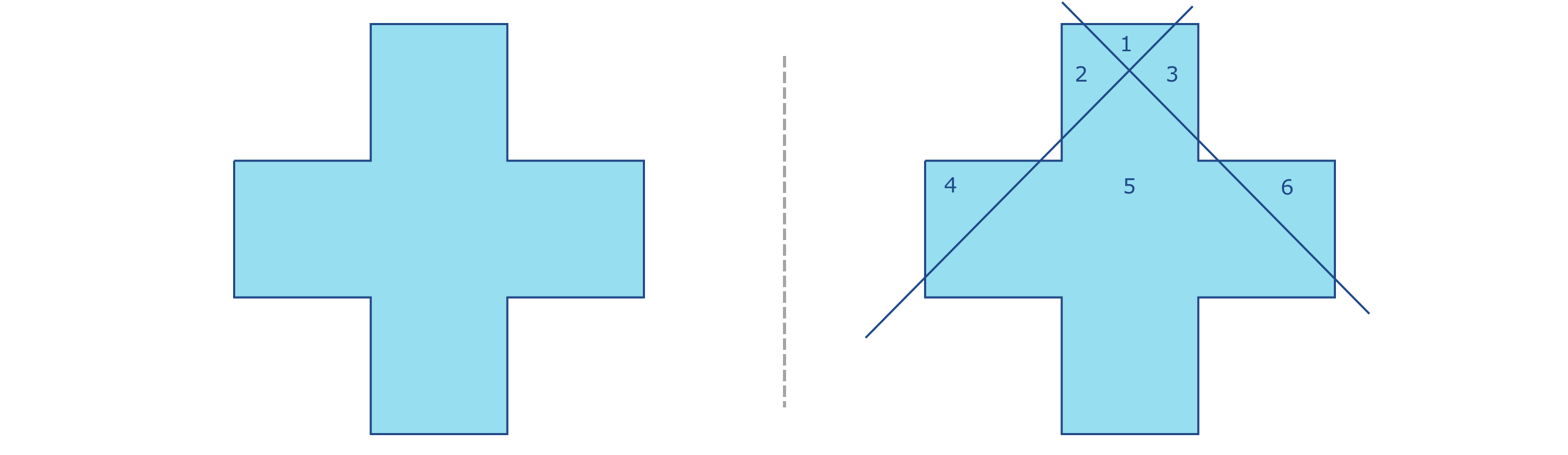George Lucas Educational FoundationiSpot / Dan Page
Brain-Based Learning

# 10 Brilliant Math Brain Teasers

Tap into rigorous problem-solving and critical thinking with these playful math brain teasers for middle and high school students.

July 28, 2023

To break the ice during the first few awkward moments of class in the new school year, high school math teacher Lorenzo Robinson uses an unusual strategy: He reads his students’ minds.

Here’s how the mystical feat unfolds: Each student picks a number between 1 and 100. Next, they use calculators to add, subtract, multiply, and divide their way through a set of predetermined numbers. At the end, everyone ends up with the same answer: 427. Robinson isn’t psychic, of course, but because the mechanics of the teaser are always the same, regardless of which initial numbers his students select, he’s able to correctly “guess” their final result—much to their amazement.

Starting off the lesson with a math brain teaser sets a playful tone and lowers the stakes for kids, “generating a buzz around my class,” Robinson says. “It makes students feel as though this class is not going to be scary, it’s going to be interesting. ‘We’re going to be learning, but we’re also going to have some fun.’”

Robinson thinks of math brain teasers as a variation on brain breaks—a brief respite from dense curricular material that gives kids time to pause and process. They can also provide an opportunity to build relationships and community as kids put their heads together to find solutions. Good brain teasers can be sneaky: They get kids developing problem-solving and critical thinking skills.

Meanwhile, before introducing a new one, Robinson works through the problem himself, identifying questions that students might have along the way and making sure his class has the background knowledge to understand how the teaser works. It’s important, he says, to provide a few minutes for kids to examine and discuss the teaser. Ask them to observe, highlight, and share things that stand out.

“The most powerful reaction is when a kid doesn’t get the correct answer,” Robinson says, and they ask to try the problem again. “They want to feel what the other kids are feeling, that educational euphoria. They want to do it again because they want to be right.” That organic intellectual curiosity is hugely helpful in high school math, Robinson says, because it can be “parlayed into the other stuff that we do.”

We combed through dozens of math brain teasers to find 10 good ones—including several of Robinson’s tried-and-true favorites.

### Number Magic: I’ll Bet Your Number is… 427

1. Start by having students pick any number between 1 and 100.
3. Multiply that number by 6.
4. Subtract 3.
5. Divide that number by 3.
6. Subtract 3 more than your original number.
8. Subtract 1 less than your original number.
9. Multiply that number by 7.

And voilà, you’ll correctly identify each student’s final result as 427.
Courtesy of: Lorenzo Robinson

1. Start with your shoe size. If you are a half size—for example, size 8.5—round up to 9.
2. Multiply your shoe size by 5.
4. Multiply that number by 20.

The first digit(s) are your shoe size, and the last two digits are your age.
Courtesy of: Lorenzo Robinson.

### Cutting Across a Cross

Ask students to draw a cross on a sheet of paper. Drawing one on the board as a point of reference is helpful. Next, ask students to draw two straight lines that will segment or cut the cross into pieces. The goal is to produce the most pieces.The solution can be found here
Sourced from: MathisFun.com.

### Number Magic: I’ll Bet Your Final Number is… 5

1. Start with a positive number. Students shouldn’t say the number out loud.
2. Square that number.
3. Add 10x the original number to what you have now.
4. Add 25 to the result of the previous step.
5. Now take the square root of that number, rounding to the nearest whole number.
7. Before students share their final figure, reveal that you guess their collective result is 5.

Courtesy of: Lorenzo Robinson.

### Birthday Math

Have students work in pairs and share the following instructions with their partner:

2. Multiply that by the month of your birth. For example, if you were born in September, you’d use the number 9 to represent your birth month.
3. Subtract 1.
4. Multiply that number by 13.
7. Multiply that number by 11.
8. Subtract the month of your birth.
9. Subtract the day of your birth.
10. Divide by 10.
11. Add 11 to that number.
12. Divide by 100.

The result on the calculator screen should be their partner’s birthday.
Sourced from: Dr. Mike’s Math Games for Kids.

### Coin Conundrum

Ask students to imagine that they have two coins that total 30 cents in value. Have them try to figure out what the two coins are, only providing them with a singular piece of information: One of the coins is not a nickel. The answer: A quarter and a nickel. (Only one of the coins is not a nickel.)

Sourced from: WeAreTeachers.

### The Phone Number Trick

1. Ignoring your area code, type the first three digits of your phone number into a calculator.
2. Multiply that number by 80.
4. Multiply that number by 250.
7. Subtract 250.
8. Divide this number by 2. Do you recognize your phone number?

Courtesy of: Lorenzo Robinson.

### A Number Challenge

For a slightly more independent teaser, challenge students to produce a math equation that works using these four numbers—2, 3, 4, and 5—as well as a plus (+) and equal sign (=). Students can work individually, in pairs, or in small groups as they try to create a valid equation. The answer: 2 + 5 = 3 + 4.

Sourced from: WeAreTeachers.

Students can work in pairs with this teaser. One person will start off by holding the calculator so their partner cannot see it; the other person can read the steps aloud to the partner with the calculator.

1. The student with the calculator starts by choosing a whole number from 1 to 20 and writing it down on a piece of paper without letting their partner see it.
2. Next, the student with the calculator enters their secret number into the calculator.
3. Multiply that number by 3.
4. Add the secret number, then subtract 5.
5. Multiply by 3, then multiply by 3 again.
6. Add the secret number, then subtract the number of their favorite month (you don’t have to know what month it is). For example, 9 represents the ninth month of the year, September.
7. Multiply by 3, then multiply by 3 again, and then again a third time.
8. Add the secret number, then subtract their favorite day of the month (again, you don’t have to know what it is on your end).
9. Ask them to show the non-calculator partner the result. At this stage, the non-calculator partner can guess the original secret number, even though what appears on the screen may be a very large number.

If the result is negative, their secret number is 1.

If the result has only three digits, their secret number is 2.

In all other cases, ignore the last three digits, and then add 2 to get the secret number!

After writing the number 8,549,176,320 on the board, ask students to observe the number and tell you everything they think is unique about the number.

Answer: It is the digits 0 to 9 in alphabetical order (eight, five, four, nine, one, seven, six, three, two, zero), but it’s surprising and fun to see what students come up with. This number can also be evenly divided by the digits 1 through 9 except for the number 7, for example.

Sourced from: MathisFun.com